### G - How many

##### Languages: C, C++, Java, Python, ... (details)

Given two sets $A$ and $B$ that have $n$ and $m$ elements respectively with $n \geq m$, determine how many ways it is possible to match all the elements so that each element of $A$ is paired with exactly one element of $B$ and each element of $B$ is paired with at least one element of $A$. Below are all the possible assignments for the first sample.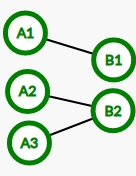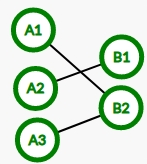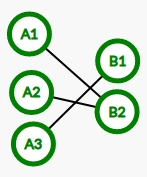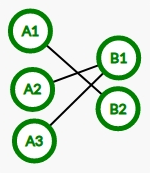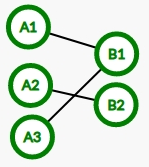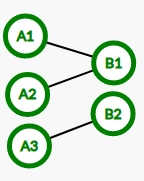#### Input

Input consists of a single line with $n$ $(1 \leq n \leq 10^{18})$ and $m$ $(1 \leq m \leq 10^5)$ separated by a space between them.

#### Output

Print the number of ways in which the elements of the sets can be matched as requested in the problem. Since this number can be quite large, print the remainder of the division of that number with prime $998244353$.

#### Sample test(s)

Input
3 2
Output
6
Input
10 3
Output
55980
Input
314159265358979323 84626
Output
683366796